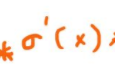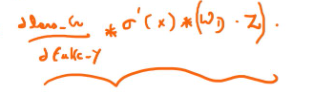The fake_Y is computed by passing the fake_X into the discriminator. Therefore when we call backward on the loss_G variable , since fake_X is computed by discriminator , will the autograd calculate the gradients of the parameter in the discriminator network ?

Yes exactly…While backpropagating since we computed fake_x through net_D , then the gradients for the net_d will also be calculated right ? But we update only net_G. Why can’t we set the gradient_req to be null for the parameters of net_D ?

`loss_G.backward()` is the only code to backpropagate.
@uniq
I’m not sure(newbie too), but I think the gradients for the net_d will also be calculated…
I’m trying to make a code note to the process.

Hi @StevenJokess and @uniq, great discussion! Since here we are given discriminator 𝐷, so we need to calculate its gradients and use it in the chain rule for the purpose of updating the weights of generator 𝐺 only. You can think of the discriminator 𝐷 as a regular function rather than a network. Let me know if it makes sense.

@goldpiggy
I agree that updating the weights of generator 𝐺 only.
But I think, the gradients for the net_d will also be calculated when updating.

OK. I found you also said that need to calculate net_D…Sorry to miss that.

discriminator D is only something that is trying to give you output ,like 0.8(near 1, so we think it is more like real data)

To calculate d(loss_G)/d(G) (G : generator network), why we need to compute the gradients of the discriminator network? The chain rule doesn’t involve any expression of the gradients of the discriminator. Correct me if i am wrong…

There is no expression involving gradients of the discriminator network. Then why we need to calculate it ?

I can’t understand what you are drawing…
Is it net_D?Sorry about my handwriting. If you write the expression for d(loss_G) / d(G) , will you find anywhere in the chain rule the expression involving gradients of D ? This is my question…Hope I am clear…If there is no expression involving gradients of D, then why we need to compute it? We can set the grad_req to be null right ?

I don’t think we still need to compute the gradients of the Discriminator network. Can you show me the chain rule expression from loss_G to the net_G involving anything with the gradients of net_D ?

This is what I am trying to convey. I still get the same loss configuration If I set the discriminator network’s parameters gradient_requirement to be null in the update_G function. Correct me If I am wrong. If the discriminator network were a large neural network , then computing the gradients will be a costly operation.

I’m not sure about my opinion.
But my thoughts are that we don’t update gradient for net_D in the update_G function, but only computing the gradient of net_D.

See the description for ‘null’. It says the gradient arrays will not be allocated. Therefore , how will the gradients will be calculated and where it will be stored ?

I guess that we just used it without storing…@uniq

" If the generator does a perfect job, then D(x′)≈1D(x′)≈1 so the above loss near 0, which results the gradients are too small to make a good progress for the discriminator. So commonly we minimize the following loss:"
Don’t you think this will lead to a large error? You can simply plot it.

I doubt about that, too.@Media @goldpiggyI guess this final expression is what the loss_G.backward() calculates, which should include net_G and net_D in the code because the gradient is calculated using the weights in net_D and net_G.

Hi, very impressive discussion about the “black box”. I wonder if any progress for GAN learning the exact matrix A and b just using the “real data”. Or maybe the NN just care about the result. That is interesting because we could have various A and b to make the data looks the “same”, howerve, they are actually different. @goldpiggy @Donald_Smith